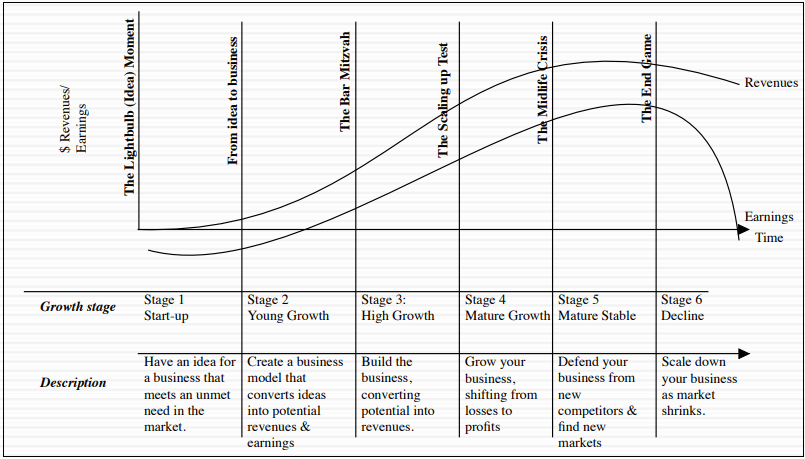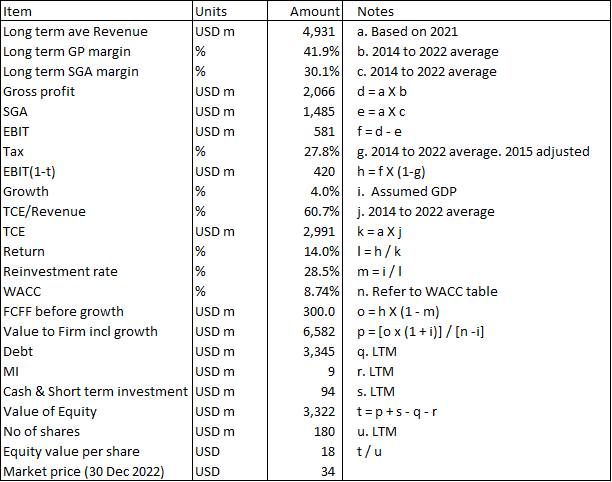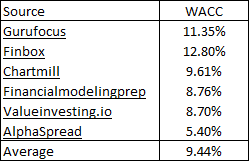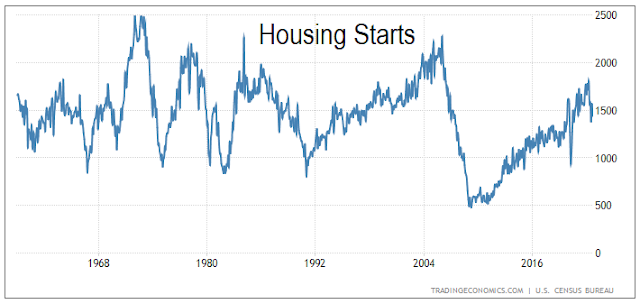### Focusing on the assumptions of perpetual growth model

Fundamentals 25: I frequently use the perpetual growth model to value companies. I called it the single-stage discounted FCFF model. This post discusses the various assumptions associated with this model. It is intended to show that there are many implicit and explicit assumptions when using this valuation model.

I am a long-term value investor looking for undervalued stocks. As such, valuation is a key element in my investment process.

My valuation approach is to determine the intrinsic value based on the free cash flow generated by the stock over its life.

To be able to do this, I have to make assumptions about the valuation model as well as the many inputs required. This is more than just assumptions about the cash flow or discount rate.

This article focuses on one particular cash flow pattern - free cash flow growing slowly over the life of the company. To value companies with such a cash flow pattern, I use the single-stage discounted Free Cash Flow to the Firm (FCFF) model. In this model growth is assumed to be the same rate in perpetuity. That is why it is called a perpetual growth model.

Join me as I discuss the various assumptions involved. I will illustrate them with examples from my steel, homebuilding, and bedding companies’ articles published in Seeking Alpha.

## Contents

• Life Cycle
• Discounted FCFF valuation model
• WACC
• Growth
• My valuation model
• Alternative EBIT approaches
• Conclusion
 This blog is reader-supported. When you buy through links in the post, the blog will earn a small commission. The payment comes from the retailer and not from you. Learn more.

## Life Cycle

All businesses go through several stages in their corporate life. Damodaran has one framework for this as illustrated in Chart 1.Chart 1: Corporate Life Cycle   Source: Damodaran

In his corporate life cycle model, there are 6 stages from Start-up to Decline.

To value a company from the Start-up to Decline stages, you need to capture the free cash flow at every stage. At the same time, you need different discount rates for each stage. This is because the discount rate is also intended to reflect the risk of the cash flow. This will differ for different stages of the life cycle.

It would be very challenging to project the cash flows and discount rates over the life of the company.

In practice, I seldom need to do this. This is because I don't invest in Start-ups and Young Growth companies. This is because I don't know how to project their cash flows. As such, I focus on the Mature ones, especially cyclical ones where historical performance can give a good indication of future performance.

The single-stage discounted FCFF model is generally applicable to companies in the Mature Stable stage. At this stage, growth is assumed to be some low and steady rate.

You will notice that my single-stage discounted FCFF model assumes that the company does not go into the Decline stage.

If you accept the corporate life cycle concept, it must mean that the values determined by my single-stage discounted FCFF model are on the high side as it ignores the Decline stage.

I generally use this model for established brick-and-mortar companies that are not facing any disruption to their business. Examples of such companies include those in the steel sector, home builders, and household products.

I am not saying that there is no product obsolescence. Rather I am assuming that such companies will continue to develop new products to replace the obsolete ones.

You can understand my concern with using this model to value tech companies where the threat of decline is common. Think of Yahoo, Nokia, and BlackBerry and you will understand what I mean.

## Discounted FCFF valuation model.

The concept here is that the intrinsic value of a company is equal to the free cash flow generated by the company over its corporate life, appropriately discounted.

You have 2 options here. The first is to consider the cash flow to just the equity holders. You then have the discounted free cash flow to equity. The cash flow here includes inflows and outflows from Debt.

The other option is to consider the cash flow from the firm perspective. You look at cash flow before interest and exclude inflows and outflows from Debt.

I use the firm approach as it is easier to handle Debt here. There are a few key ideas behind this model:
• Discounting to account for both the time value of money and risk.
• Using FCFF as the cash flow.
• Differentiating between operating and non-operating assets.

• The value of the firm accrues to all providers of capital.

### Discounting

For the single-stage discounted FCFF valuation model, the value can be represented by the equation

Value = FCFF × (1+g) / (r-g).

Where:

FCFF = the Free Cash Flow to the Firm for the base year.

r = Discount rate = WACC.

It is referred to as the single-stage model because there is only one growth rate and one discount rate. It assumes that the FCFF increases annually by the same "g". It also assumes that the discount rate for the various periods are multiples of the same base WACC.

Note that the above model is a formula derived from the following present value equation.

You can see that it assumes the same growth rate and discount rate for all the years. It also assumes that the growth rate is an annual one. In other words, it increases once a year.

More importantly, the model assumes that the base year FCFF together with the growth rate is a good representation of the future cash flows.

Because of this, I spend considerable effort establishing the base FCFF. Sometimes I use the latest value. Sometimes I use the normalized value.

### FCFF

The FCFF can be thought of as the after-tax cash flow available to the providers of capital after accounting for CAPEX and increases in working capital. This is similar to what Warren Buffet calls "owners’ earnings". The "owners" here refer to all the providers of funds used by the company.

In practice, this FCFF is derived by first determining the earnings before interest and taxes (EBIT). You then act as if you paid taxes on the EBIT even though in real life, companies pay taxes after deducting interest charges.

You thus get the amount EBIT × (1-t) that is commonly written as EBIT(1-t) where "t" represents the tax rate. You next deduct any CAPEX and increases in Working Capital and add back Depreciation & Amortization.

Mathematically the FCFF can be represented by:

EBIT(1- t) - CAPEX - NWC + D&A.

Where:

CAPEX = Capital expenditure.

NWC = Increases in non-cash Working Capital.

D&A = Depreciation and Amortization.

The term CAPEX + NWC - D&A is referred to as Reinvestment.

And Reinvestment rate as denoted by Re = Reinvestment/EBIT(1 - t).

The FCFF can then be represented by EBIT(1-t) x (1-Re).

### Operating and non-operating assets.

EBIT is generated by the operating assets.

Examples of operating assets are plant & machinery and net working capital. It excludes cash, investment in associates, and investment in securities as these are generally not considered part of the normal business operations.

Given this, the intrinsic value of the firm = Value of operating assets + Value of non-operating assets.

In other words, you have to add the value of cash and other non-operating assets to the value obtained from discounting the FCFF.

This would require you to determine the values of the various non-operating assets. For example, for investments in other companies, you should use the appropriate portion of the intrinsic values of these companies.

In practice, I take cash and other non-operating assets at their Book Values. I assumed that they are small components of the total intrinsic value. Determining the intrinsic value of these non-operating assets would not make a big difference to the total intrinsic value.

For example, in my valuation of Tempur- Sealy, I determined the value of the operating assets as UDS 6,852 million - item “p” in Table 1.

I then added the non-operating assets – item “s” in Table 1.

But I have seen arguments that if companies have a bad track record in deploying cash, there should be a discount to the Book Value. This is especially if the cash component is significant.Table 1: Estimating the Value of Tempur-Sealy

### Value to all providers of capital.

The value obtained by adding the operating assets and non-operating assets belongs to all the providers of funds.

To determine the value to the equity holders you have to deduct what is due to the other providers of funds. These are the portion due to the minority shareholders and those providing Debt.

Technically Debt should be based on the market value of Debt rather than the Book Value. There are theoretical ways to determine the market value of Debt but this is a story for another day. In practice, if the amount is small eg DE ratio less than 1, I assumed the Book Value.

Similarly, I also use the Book Value when considering the value of the Minority Interests.

I referred to the Tempur-Sealy valuation earlier. To derive the Value of Equity, I deducted the Debt and Minority Interest. This resulted in USD 3.322 – item “t” in Table 1.

You can see that assumptions are made from the start - the stages of the life cycle, Value of non-operating assets, Value of Debt, and Value of Minority Interests. This is even before considering parameters used to determine the value of the operating assets.

## WACC

Because I am looking at the cash flows to the firm, the discount rate used is that for the firm. This is commonly called the weighted average cost of capital (WACC) as it takes into account the cost of equity as well as the cost of Debt.

The cost of equity is derived from the Capital Asset Pricing Model (CAPM). This requires assumptions about the risk-free rate, the risk premium, and the risk.

Risk in this context is measured by the Beta of the firm. In other words, you assumed that volatility = risk.

The cost of Debt is based on viewing all the Debt as equal to a single bond. It is not the Book Value of Debt.

According to Investopedia, one way to calculate the after-tax cost of Debt = (risk-free rate + credit spread) × (1-t).

You will appreciate that there are assumptions to derive the cost of Debt. Damodaran has a good write-up on the WACC. Refer to “The weighted average cost of capital – NYU Stern

In practice, I sometimes derive the WACC following Damodaran's approach. But for US companies there is a lot of readily available information. As such I used the average value based on a Google search of the WACC.

A good example is how I derived the WACC for the building materials distributor BlueLinx as shown in Table 2.

The key point is that there are several assumptions in estimating the WACC. As such, there is no one figure for the WACC.Table 2: Estimating the WACC for BlueLinx

## Growth

The underlying assumption here is that the long-term growth rate will be less than the discount rate. Next, growth is assumed to be the same in perpetuity.

For this to be realistic, the growth rate has to be smaller than the GDP growth rate. Damodaran has even suggested that it should be the risk-free rate.

The first thing then is to check that the assumed growth rate is less than the GDP growth rate. The question then is what to compare with. Do we use revenue, earnings, or assets?

If you believe that growth is tied to fundamentals then we should compare it with earnings and capital employed. This is because growth is linked to fundamentals by the equation:

Growth rate = Return × Reinvestment rate.

Given the growth rate, we can then determine the Reinvestment rate (Re) = Growth rate/Return.

In the context of the Firm, Return = EBIT(1-t)/TCE where:

TCE = Total Capital Employed = Shareholders Funds + Minority Interests + Debt - Cash & Other Non-operating Assets.

For consistency, the TCE should be the capital employed to generate the EBIT.

Once we know the Return, we can determine the Reinvestment rate. In turn, we can derive the FCFF from the equation

FCFF = EBIT(1-t) × (1- Re).

The challenge here is determining the TCE. To estimate the TCE, I first determine the average TCE/Revenue ratio for the company.

With this ratio, I then determine the TCE for the projected Revenue. We can then use this to derive the corresponding Return. Thereafter we can derive the Reinvestment rate.

The key assumption here is that the Reinvestment rate is constant. In many cases, you will find that the actual historical Reinvestment rate differs from the derived rate.

This is partly because CAPEX is a lumpy expenditure in real life. Secondly, management has some discretion in managing working capital.

The other assumption is that there is some fixed ratio between TCE and Revenue.

The TCE/Revenue ratio is similar to the inverse of the Asset Turnover. In the DuPont analysis, there is a link between the ROE and Asset Turnover. We can imagine a similar relationship between the Return defined here and the TCE turnover.

If we assumed that the Return is stable, then the TCE/Revenue ratio should also be stable. In principle, you can also determine the FCFF by deducting the Reinvestment items from EBIT(1-t).

I have carried out valuations where I first determined the actual FCFF for every year. I then determined the base FCFF as the average FCFF of the various years.

You should not be surprised that the base FCFF derived from this average method is different from that derived based on EBIT(1-t) x (1-Re).

Which is more appropriate? I don't think there is an answer. Each is based on a different set of assumptions.

I prefer to use the one based on EBIT(1-t) × (1-Re) because I can link the growth rate to EBIT. If I use the average FCFF approach, I would have to link the growth rate to the growth in the FCFF.

 Case NotesAccording to the Management Study Guide,The FCFF valuation model is almost analogous to the Gordon model except that it uses other components as inputs to the calculation.But there are some differences between them:The single-stage FCFF model uses Free cash flow to the firm as an input whereas the Gordon growth model uses dividends as an input.The single-stage FCFF model discounts the cash flows with the WACC. The Gordon model discounts the dividends with the cost of equity.The single-stage FCFF model has to make adjustments to include the after-tax cost of debt while calculating the weighted average cost of capital.Many models can be used to value companies. As a newbie, you can be overwhelmed by the many choices. My advice is to use the simplest ones given the many assumptions required. An alternative is to look at what others have done. Sites like Seeking Alpha* are good sources for such information. Click the link for some free stock examples. If you subscribe to their services, you can tap into their business analysis and valuation.

## My valuation model

Table 1 illustrates how I computed the intrinsic value based on the single-stage discounted FCFF. It takes into account the value of the operating and non-operating assets. It deducts Debt and minority interests to get to the value to the equity holders.

The starting point in my valuation model is how I derive the FCFF. This in turn depends on how I derive the EBIT.

The assumptions used to derive the EBIT are generally tied to the company's characteristics. This will differ from case to case. As such I will just cover the general assumptions in this post.

### EBIT

There are several ways to derive the EBIT. In the model shown in Table 1, the EBIT was derived based on Gross Profit less SGA expenses. Refer to item “f” in Table 1.

Gross Profit = Revenue × Gross Profit margin

SGA = Revenue × SGA margin

The Revenue, Gross Profit margin, and SGA margin are "normalized" values. How I derived the "normalized" values would depend on the company. In the Tempur-Sealy case as per Table 1, the normalized values were based on:
• The current revenue. This is to reflect the current size of the company.
• The normalized gross profit margins and SGA margins. These were based on the values over the cycle. The cycle period was assumed to be from 2014 to 2022 based on the Housing Starts cycle.

In the case of Housing Starts, the actual peak-to-peak was 2005 to 2022 as shown in Chart 2. But unfortunately, Tempur-Sealy in its current form came into the picture in 2013. Thus, the only data available for determining the average values was from 2014 to 2022. The assumption is that this period is a good representation of the cycle.Chart 2: US Housing Starts.   Source: Trading Economics.com

As can be seen, the starting point for my valuation was Revenue. The value for this metric will depend on the situation.

In my Steel Dynamics analysis, I determined Revenue by looking at the shipping tonnage and then relating this to the long-term average revenue per ton. Steel is a cyclical commodity and while unit prices vary widely throughout the cycle, the shipment quantity is more "stable". Growth comes from the shipment tonnage rather than the cyclical prices.

In my Boise Cascade analysis, I pegged Revenue to the long-term average annual Housing Starts. This is because while House Starts are cyclical, there is no growth in the long-term average annual Housing Starts of about 1.5 million units. The Revenue is for the year when the Housing Starts was 1.5 million units.

The underlying assumption here is that the margins are stable within the range of Revenue considered. We know that certain items in the cost of sales eg depreciation and staff costs do not vary per unit of output. Rather they have stepped changes. But we act like they are continuous variables. This is a reasonable assumption if the volume is far above the breakeven level.

### Tax rate

According to Damodaran, we should use the nominal tax rate rather than some average of the actual tax rate. This is because we are taking a long-term view whereas actual tax rates may be affected by short-term tax adjustments.

Using a nominal rate works if the company operates in only one country. But if the company operates in several countries with different tax codes, I find it difficult to determine a nominal rate. In such cases, I used the actual average tax rate.

In practice, changes in the tax rate are not as significant as changes in the FCFF or WACC on the intrinsic value.

### SGA

Selling, general and administrative expenses generally have step changes. Even if you consider them variable, they should only apply to a particular Revenue range.

In the example shown in Table 1, I have assumed that the SGA is a variable linked to the Revenue. That is why I used the SGA margin.

## Alternative EBIT approaches

The model illustrated in Table 1 is not the only way to determine the base year EBIT or normalized EBIT. The template shown in Table 1 is a general one. But there are other ways involving different assumptions as illustrated by the following.

In my Leggett & Platt analysis, the EBIT was based on the EBIT/Total Asset ratio. The growth was then the growth in Total Assets. This was because it was difficult to estimate the Gross Profit margin or SGA margin. Refer to “Leggett & Platt: Limited Financial Benefits From ECS Acquisition

In the case of Stelco, I used the average EBITDA and then deduct Depreciation & Amortization to derive the EBIT. In this case study, there was not enough information to derive the Gross Profit margin. Refer to “Stelco Holdings: Grossly Underpriced Cyclical Growth Company

In the TMST analysis, I estimated the Gross Profit for the base year directly by taking the average Gross profit. I then deducted SGA to arrive at the EBIT. This was because the company was operating below breakeven levels for most of the period. For the base year, I wanted the intrinsic value if it operated above this breakeven level. Refer to “TimkenSteel: Under-Priced Even Through A Cyclical Lens

## Conclusion

All valuations are based on assumptions. There are several types of assumptions involved:
• The valuation model used.
• The values of the non-operating assets.
• The values of Debt, Minority Interest, and other non-equity provider of funds.
• The values of the key parameters to determine the FCFF.

The point is that it is not just the assumptions to derive the FCFF. As shown in this article there are other assumptions. With so many assumptions you could be forgiven for doubting the accuracy of the value obtained.

I agree. That is why we have a margin of safety.

Given the many assumptions you may also wonder why to bother with the DCF method when relative valuation is much simpler. However, when you use relative valuation, you are pretending that there is no assumption. In reality, the assumptions are implicit.

With the single-stage discounted FCFF model, the assumptions are explicit and can be easily challenged. I rather know that they are based on assumptions than not knowing what has been assumed as with the relative valuation method.

In valuing companies, we make many choices and the intrinsic value obtained reflects the choices made. That is why different people will get different intrinsic values even though they all use the same single-stage discounted FCFF approach.

Because of the assumptions, I use 3 valuation approaches to triangulate the intrinsic value of a stock. They are Asset-based valuation, Earnings-based, and the Acquirer Multiple.